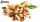Playing

How long have we trained on the pitch when we know that the warm-up took 10 minutes, we trained passes for one-third of the time and we played football half the time?

Result

t =  60 min

Solution:

10 + t/3 + t/2 = t

t = 60

t = 60

Calculated by our simple equation calculator.

Leave us a comment of this math problem and its solution (i.e. if it is still somewhat unclear...):Be the first to comment!To solve this verbal math problem are needed these knowledge from mathematics:

Need help calculate sum, simplify or multiply fractions? Try our fraction calculator. Do you have a linear equation or system of equations and looking for its solution? Or do you have quadratic equation?

Next similar math problems:

1. Cleaning windowsCleaning company has to wash all the windows of the school. The first day washes one-sixth of the windows of the school, the next day three more windows than the first day and the remaining 18 windows washes on the third day. Calculate how many windows ha
2. Unknown numberIdentify unknown number which 1/5 is 40 greater than one tenth of that number.
3. PearsThere were pears in the basket, I took two-fifths of them, and left six in the basket. How many pears did I take?
4. UN 1If we add to an unknown number his quarter, we get 210. Identify unknown number.
5. Six yearsIn six years Jan will be twice as old as he was six years ago. How old is he?
6. Equation with xSolve the following equation: 2x- (8x + 1) - (x + 2) / 5 = 9
7. Simple equation 6Solve equation with one variable: X/2+X/3+X/4=X+4
8. EqnSolve equation with fractions: 2x/3-50=40+x/4
9. EquationSolve the equation: 1/2-2/8 = 1/10; Write the result as a decimal number.
10. One thirdIf 3/5 is 360, how much is 1/3?
11. Simple equationSolve for x: 3(x + 2) = x - 18
12. Unknown numberI think the number - its sixth is 3 smaller than its third.
13. Bag of peanutsJoe eat 1/3 of a bag of peanuts, mark eat 1/4 of the remaining in the bag of peanuts, Alvin eat 1/2 of the remaining bag of peanuts, peter eat 10 peanuts, there are 71 peanuts left. Hon many peanuts were in the bags?
14. Eq-fracSolve the following equation with fractions: h + 1/3 =5/3
15. Equation with fractionsSolve equation: ? It is equation with fractions.
16. FractionsThree-quarters of an unknown number are 4/5. What is 5/6 of this unknown number?
17. Fraction + eqSolve following simple equation with fractions: -5/6(8+5b) = 75 + 5/3b